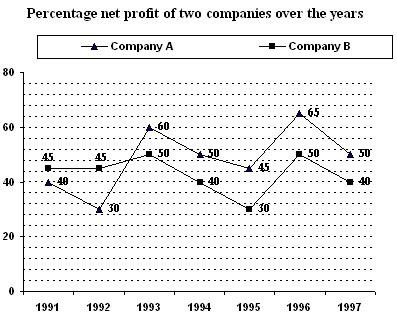### Data Interpretation for IBPS Exams 2017

Direction (01-05): Study the following graph carefully and answer the questions given below:1). If the total income in 1991 for Company B was 140 crores, what was the total expenditure in that year?
a) 100 crores
b) 110 crores
c) 98 crores
e) None of these

2). If the total expenditure of 1993 and 1994 together of Company B was Rs.270crores, what was the total income in these years?
a) Rs.121.5 crores
b) Rs.135 crores
c) Rs.140 crores
e) None of these

3). In how many of the given years the percentage of expenditure to the income of Company A was less than fifty?
a) One
b) Two
c) Three
d) Four
e) None of these

4). If the total expenditure of company B in 1994 was Rs.200 crores, what was the total income?
a) Rs.160 crores
b) Rs.240 crores
c) Rs.260 crores
e) None of these

5). In which of the following years was the total income more than double the total expenditure in that year for Company B?
a) 1995
b) 1993
c) 1997
d) 1992
e) None of these

Direction (06-10): Study the chart and give the answer of following questions.

6). 50% of all the cars consisted of which colours of car?
a) Black, Golden, Blue, Red
b) Blue, Black, Red, Silver
c) White, Golden, Blue, Black
d) White, Blue, Green, Black
e) None of these

7). Cars of which colour are 20% less popular than white coloured cars?
a) Black
b) Golden
c) Red
d) Blue
e) None of these

8). Cars of which colour are 13% less popular than white cars?
a) Blue
b) Green
c) Silver
d) Yellow
e) None of these

9). Cars of which colour when increased by two per cent and then combined with that of red cars will make 30 per cent of the total?
a) Golden
b) Blue
c) Black
d) Yellow
e) None of these

10). If in a certain period the total production of all cars was 95400 then how many more blue cars were sold than green?
a) 2580
b) 3618
c) 2850
d) 3816
e) None of these

Solution:

1). According to Questions 145% à 140 crores
Therefore 100% à ? = 140/145 ×100 = 96.55 crores

2). Let the expenditure of company B in 1993 is Rs.x and 1994 is Rs.y
According to question = 50% of x + 40% of y = Rs.270 crores
0.50x + 0.40y = Rs.270 crores
Here, the number of equations available is one and number of unknown quantities available is two. Therefore, we can’t determine the required answer.

3). It is clear from the graph.

4). Required answer = 40% of 200 = Rs.80 crores

6). Required answer = 26 + 6 + 13 + 5 = 50%

7). Required answer = 26% – 20% = 6%

8). Required answer = 26% - 13% = 13%